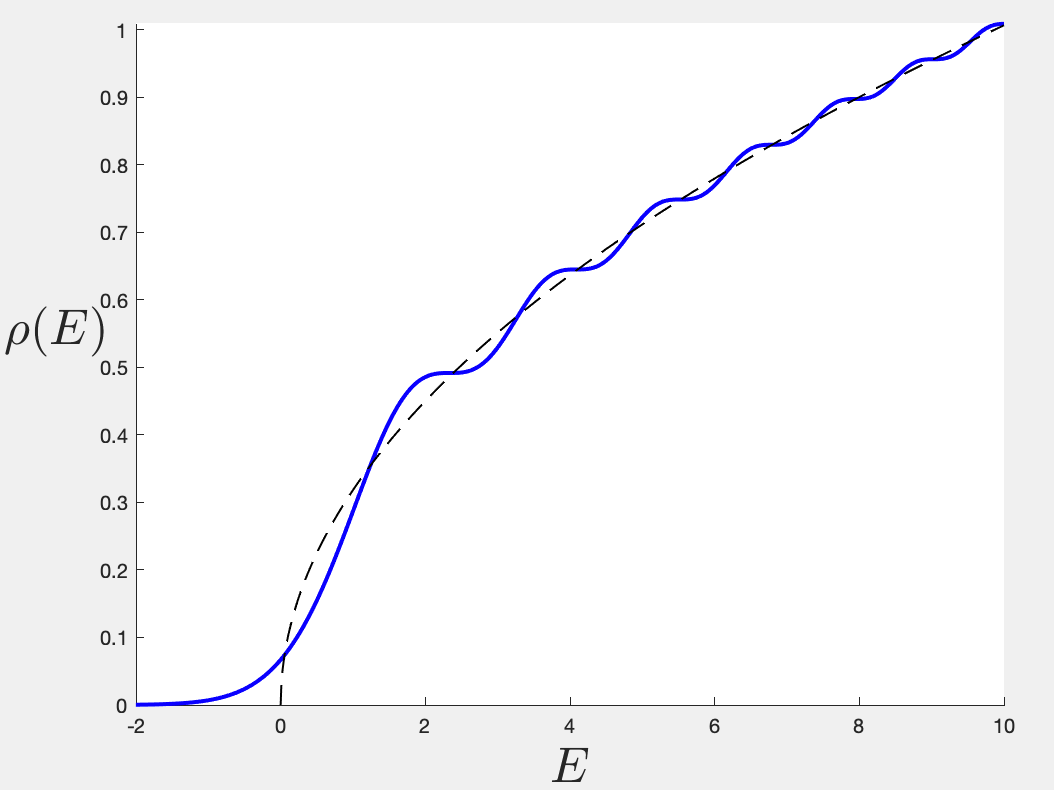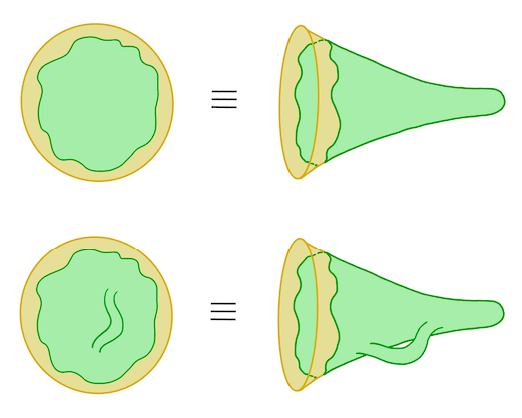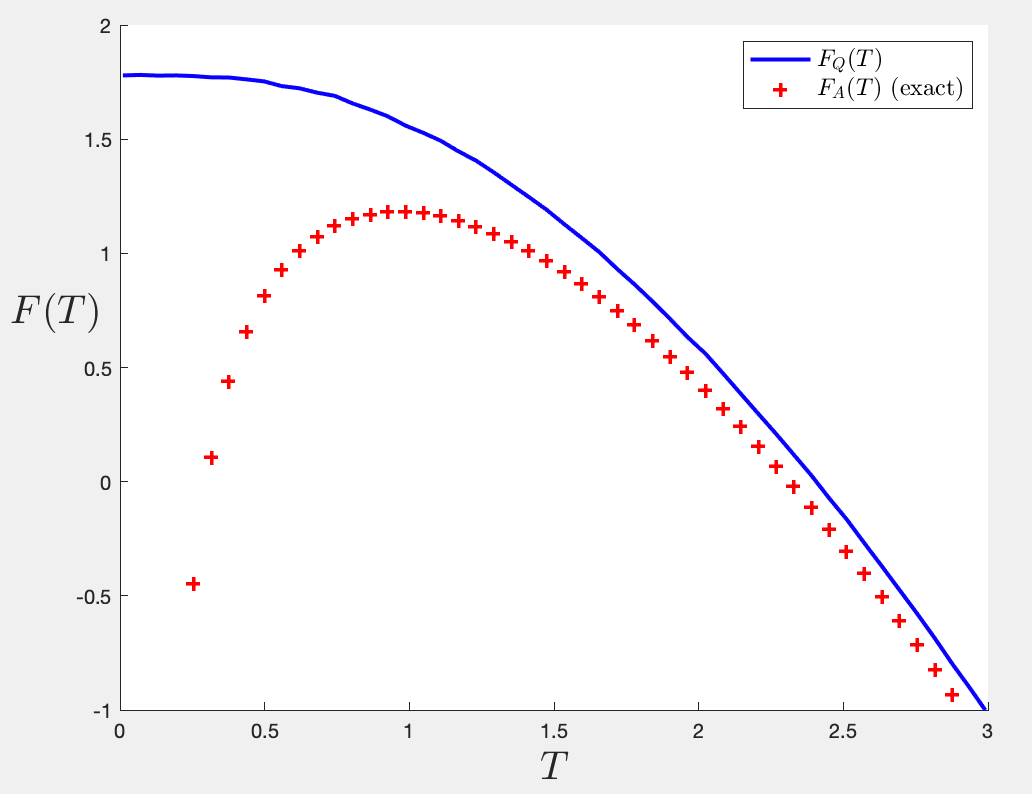# Matrices and Gravity

[A somewhat more technical post follows.]So I have a confession to make. I started working on random matrix models (the large$N$, double-scaled variety) in 1990 or 1991, so about 30 years ago, give or take. I’ve written many papers on the topic, some of which people have even read. A subset of those have even been cited from time to time. So I’m supposed to be some kind of expert. I’ve written extensively about them here (search for matrix models and see what comes up), including posts on how exciting they are for understanding aspects of quantum gravity and black holes. So you’d think that I’d actually done the obvious thing right? Actually taken a bunch of random matrices and played with them directly. I don’t mean the fancy path integral formulation we all learn, where you take N large, find saddle points, solve for the Wigner semi-circle law that the Dyson gas of eigenvalues forms, and so forth. I don’t mean the Feynman expansion of that same path integral, and identify (following ‘t Hooft) their topology with a tessellation of random 2D surfaces. I don’t mean the decomposition into orthogonal polynomials, the rewriting of the whole problem at large$N$ as a theory of quantum mechanics, and so forth. No, those things I know well. I just mean do what it says on the packet: close your eyes, grab a matrix out of the bag at random, compute its eigenvalues. Then do it again. Repeat a few thousand times and see that all those things in the data that we compute those fancy ways really are true. I realized the other day that in 30 years I’d never actually done that, and (motivated by the desire to make a simple visual illustration of a point) I decided to do it, and it opened up some wonderful vistas.

Let me tell you a little more. You’re going to have to do some of the work and look at earlier things I’ve written for some of the background so that I don’t have to repeat myself. The starting point may well be my pair of posts entitled “Black Holes and a return to 2D Gravity”, Part I and Part II. There you will learn a bit about how the two-dimensional gravity theory called JT gravity is potentially useful for understanding the low temperature dynamics of more grown-up near-extreme black holes in (e.g.) the four dimensions we live in. Those dynamics are actually quantum dynamics. In other words, we’re learning something about a quantum description of spacetime physics that is close to what we care about in our world. (In brief: the zero temp 4D physics factors into a 2D gravity (AdS2) times a fixed two-sphere. Moving away from that limit is captured by the 2D theory known as JT gravity.)

Anyway, I’ve been trying to get people to understand and appreciate more why we should all be excited about the matrix model description of JT gravity (and hence near extremal 4D dimensional black holes), particularly when you can get access to what I’ve called “non-perturbative physics”. Perturbation theory is a topological expansion in the genus or the 2D gravity surfaces here: They all have one boundary (periodic Euclidean time) and so you start with the disc and add handles, and possibly more boundaries, to compute your various physical results. But non-perturbative physics uncovers some very important features. Among those are the “bumps” in the spectral density of the theory. Here’s an example for a toy model called the Airy model (where the answer can be written analytically).Full spectral density for the Airy model. Dotted line is leading perturbative part.In the figure, the dotted line is the leading perturbative piece – the disc order – which is$\rho_0(E)=\sqrt{E}/(\pi\exp(-S_0))$. At the next order would be a disc with one handle (see figures on the right), and the result is$-\frac{7}{48\pi}\exp(-S_0)$, and so on. Then there are corrections that can’t be written as a series expansion in$\exp(-S_0)$, the `non-perturbative’ parts that make up the full$\rho(E)$, which is given in blue. You can’t get those bumps from perturbation theory. Once you have the full thing (in the example above it can be written in closed form in terms of Airy functions) then by Laplace transform you have the full partition function$Z(\beta)=\int \rho(E) e^{-\beta E} dE$. (Here$\beta\equiv1/T$, and I’ll go back and forth between the two parameters.)

Well, those bumps are a sign of the underlying discreteness of the spectrum, and in particular, matrix model statistics is such that eigenvalues don’t like to lie near each other (in fact they repel) and so they spread out, taking up positions are on average nicely spaced from each other in any typical spectrum. My main point is that the map between matrix models and JT gravity, and JT gravity and near-extreme black holes, identifies the matrix eigenvalues with the black hole quantum micro-states.

Putting it more quantitively, recall that the topological expansion parameter,$1/N$, of the matrix model gets identified with the$\exp(-S_0)$ of the gravity theory, where$S_0$ is the extremal entropy of the black hole. Taking a log of both sides you see that$S_0=\log N$, which is just the Boltzmann expression for the entropy in terms of the number of micro-states. That’s nice. So whatever we learn about (double-scaled) random matrix model states should be taken as lessons about black hole micro-states. At least, that’s the main lesson I took away from the original Saad, Stanford, and Shenker paper showing that JT gravity has a matrix model description, which is one of the reasons why I’ve been thinking about the non-perturbative physics of it. That’s at the heart of the quantum gravity guts of the black hole story.

So anyway, to cut a long story short, much of my research last year was showing how to actually formulate the matrix model descriptions of several JT gravity variants such that one can actually uncover those bumps in the spectrum. And I succeeded in doing it for a variety of examples. (You can look here or at its companion – the pair were an editor’s suggestion in PRD, which was nice.) Many interesting features of the various results struck me as interesting, and so the natural question has been how best to understand the physics of these underlying micro-states that are revealing themselves? In particular, how does the thermodynamical description of the black holes, that we are pretty used to at high temperatures, extend down to lower temperatures when we must understand it in terms of the micro-states and their statistical properties?

It is these questions that have been on my mind for a while, and the big specific question I’ve been asking (as have others, like Engelhardt et. al.) is: “How do you compute the quenched free energy of JT gravity?”. This is the quantity$F_Q(T)=-T\langle \log Z(T)\rangle$, where$Z(T)$ is the partition function of the theory. The free energy we usually calculate in gravity is what stat mech people would call the annealed free energy,$F_A(T)=-T \log \langle Z(T)\rangle$, which is easier to calculate typically because you usually compute things using a path integral and the basic object to be defined is usually$Z(T)$ and expectation values and averages of products thereof, while the same things are hard to compute for the logarithm of it. I won’t go deeply into it here, but you can find lots of references online or in textbooks on statistical physics. The point is that$F_Q(T)$ is arguably the thing you really want to compute in order to extract things like entropy$S(T)$, etc., of the system. If you stick with$F_A(T)$ for all temperatures, you’ll run into problems. Let me show you the graph of$F_A(T)$ for the simple toy model (the Airy model):

This just comes from working out that$Z(T)$ from before and taking the logarithm and multiplying by$-T$. You can see that, even with all the non-perturbative information included, as temperature decreases problems develop, as can be seen from working out the entropy (above the extremal$S_0$), which is just (-) the slope. It goes negative (bad), and also diverges (also bad). This is not good. (For those who have done their homework, the same thing happens in any generic JT gravity theory… this is nothing to do with special properties of the Airy model or anything like that.) What you really want is$F_Q(T)$, which will agree with$F_A(T)$ at high temp, but the two will deviate at lower temp and$F_Q(T)$ will presumably (hopefully!) be well-behaved all the way down to$T=0$.

Well, my point, since a paper I wrote last August, is that the answer to the question about how to compute$F_Q(T)$ for JT gravity (and variants thereof) should be (must be?) that you use the matrix model description. The logic is simple: The matrix model tells you about how to compute non-perturbative effects in the gravity theory, and those are most important at low energy, so if you don’t have such a tool to uncover the role of those things, you’re not uncovering the whole story about the free energy. I took a first pass at it in the August paper for a very simple model and got a partial hint of how it all ought to work (showing that indeed non-perturbative features of the density do control the leading low temperature behaviour of$F_Q(T)$), but it was an partial argument on a simplified model, so more work needed to be done. I wrote (version 1 of) a paper a couple of weeks ago, that had some nice results, but the final story was not as clean as I’d hoped, and the relation to recent other work on the low T physics (by Janssen et al) was obscure to me. (Since I was working on my paper using a very different method, due to Okuyama, I hadn’t really taken the time to properly understand what they’d done). You’ll see below how that all changed.

But in any case, here’s my confession. Having finished the paper, I decided to take some time out from specific project work for a day or two, and decided to play (perhaps to some this is an odd bit of entertainment) with some random matrices for real, and build a concrete illustration of the often-said words (above) about the bumps in the spectral density showing properties of the underlying discrete spectrum. The idea is that an individual matrix would have a series of delta functions at the energies in the spectrum, but a given matrix randomly chosen might have its energies lightly to the left or the right of those of another member of the ensemble, so the delta-function spikes broaden out into peaks if you put all the spectra together. See this for real meant, as I said, that I sat down and generated random (Gaussian) 100 by 100 Hermitian matrices, (a couple of lines of code in MatLab) and worked out their eigenvalues (one line of code), and repeated thousands of times. Bin the eigenvalues with histograms and the famous Wigner semi-circle emerges quite readily for the shape of the distribution (takes less than a minute). Embarrassingly, I’ve never explicitly done this before! The next step was to focus attention on an endpoint of the distribution, and focus attention there while taking the scaling limit on the energies (zoom in by a power of$N$ as you send it large) that the double-scaling limit dictates. So rescale all your binned results to suit. Now when running over samples, if you take care to keep track of the order of the energies in each spectrum, you can also bin data (and then plot histograms) of what the lowest, next lowest, next next lowest, etc., energies were. Noodling around with some numbers of order one to match your overall scaling, and it lines up with the exact result I showed earlier, computed using the fancy methods, viola!

There’s the famous “leaking out” of the eigenvalues from the semicircle edge into the negative$E$ region, now demonstrated with real occurrences that happened, and so on. The first peak is very famous. It is the Tracy-Widom distribution law (usually quoted for the largest eigenvalue, but it applies equally to the lowest in this case). It shows up (for mysterious reasons) in a wide variety of random problems. (Look here for example.) There’s a blue dashed line showing the full solution that comes from an analysis involving lots of fun things like Fredholm determinants, Airy kernels, and the Painleve II equation (which I solved numerically), for illustration. Note that the average energy (which here is the average energy of the ground state) is$\langle E_0\rangle \simeq 1.77$.

So anyway, that’s my confession. I’d never done that hands-on exercise of sampling matrices before and it is so simple and (although I knew it “intellectually”) somehow illuminating. (The nature of writing online is that there’s going to be one or two readers already typing snotty remarks into the comments about how well-known and trivial this is. Good for them, those advanced beings. I never said it was hard or unknown, just nice to do.) I’ve had other experienced matrix model researchers tell me they also found it illuminating to simply look at these results. Sometimes we get so into the sophisticated methods that we forget to get our hands dirty with the basics.

So, well, this was such fun that I continued. You can make one change in the short computer program to build Hermitian matrices that have a positive spectrum (“Wishart” matrices they are often called:$H=MM^\dagger$) and the emerging models in the scaling limit will be a well-known class of “Bessel” models. These (yep, they are built out of Bessel functions) are very important in JT gravity and JT supergravity too, so insights are very useful there too. So I did the same sampling job there. Here’s an example:

So here’s the punchline. A short while after feeling pleased with myself for the success of this exercise in illustration, I realized that I could do an obvious thing. Since the results line up so nicely from scaling and sampling 100 by 100 matrices, why don’t I just mine the same data set and compute the quenched free energy directly this way?! So obvious. Why had I not thought of this before? (It seems that from the literature, nobody had.) This takes another five minutes to add the right lines of code, and then run a loop to do it over several sample temperatures, and voila!The quenched free energy for the Airy model vs the annealed. The entropy goes nicely to zero!

Some nice things to notice:

• The quenched free energy indeed asymptotes to the annealed free energy at large temperature.
• The entropy (minus the slope of the blue curve) runs nicely to zero at zero temperature, where indeed all that is left is the extremal entropy$S_0$. It’s having nothing to do with the negativity and divergence you get from the red curve.
• The free energy lands at a special value at zero temperature:$\sim 1.77$, the average value of the ground state energy. This is nice and also reasonable! The influence of the micro-physics is present in a very natural way!
• It turns out (and this is when I properly began to appreciate the lovely analysis of a recent paper by Janssen and Mirbabayi who did some low temp predictions of this) that the initial fall off from zero temperature looks rather flat, and this is because it goes like$-T^4$, with a coefficient that is again controlled by the underlying statistics of the microphysics (the distribution of the gap$E_1-E_0$ in fact, worked out by Forrester, Bornemann and Witte, and by Perret and Schehr (References in my paper)). Of course, this way you get more than just the low temperature limit, you get the whole thing.

How lovely to see the whole behaviour for arbitrary temperature come out so nicely!

I won’t go into it here, but this is just the beginning. That large family of Bessel models I described has several nice phenomena that can be studied analytically and laid alongside the numerics. There’s even more wonderful results in the statistical mechanics literature that then show up in the analogous computation of the quenched free energy (that again does not seem to have been done before). For example, the first peak (red) in the Bessel example above turns out to be an exponential (Edelman, Forrester), and the average energy is simply$\langle E_0 \rangle =4$, an exact result. Here’s that showing up nicely in my quenched free energy result:

I could go on about other features, and the numerous other exciting results I got by mining this rich seam of models, but I’ll instead refer you to the paper. (In case you already looked at it, note that there’s a lot of new material (all of this and more) in version 2, and it has a major correction to the overall picture that was painted in v1. It also has a big shout-out to Janssen and Mirbabayi’s very nice recent low T analysis that a lot of my results verify and extend.)

So here’s the big picture thought to wrap up all of this. What was shown above extends to the full matrix models of JT gravity (if you use a stable definition) and variants thereof. (Although it is (so far) hard to generate accurate explicit curves of the sort I showed above for the toy models, see the paper for more on that). I keep emphasizing that this is a fantastic example of the quantum black holes’ (i.e., 4D extremal black holes’) explicit microphysics at play here. The detailed behaviour of the free energy, including its eventual zero temperature value, is all controlled by this microphysics. Without having control of the microphysics in full detail (e.g. by using the matrix model description as I’ve done here, or something equivalent), these features can’t be derived.

What fun!

-cvj